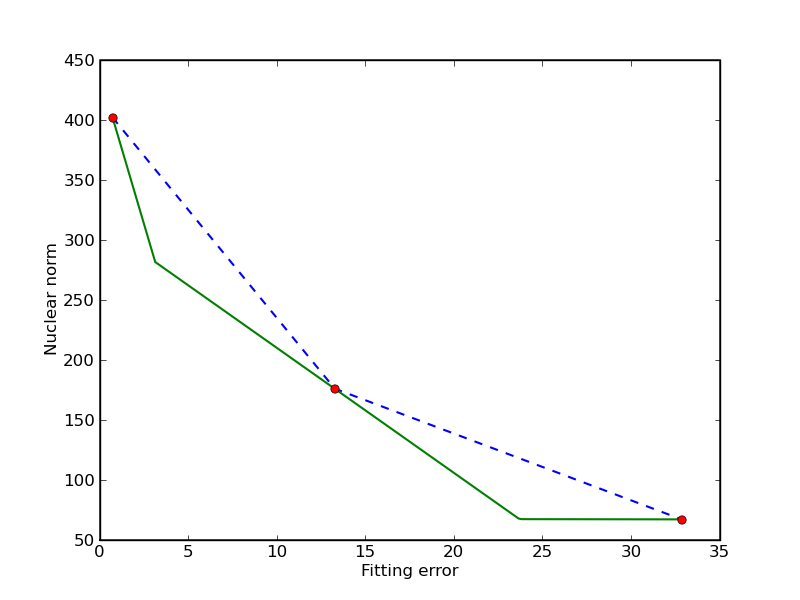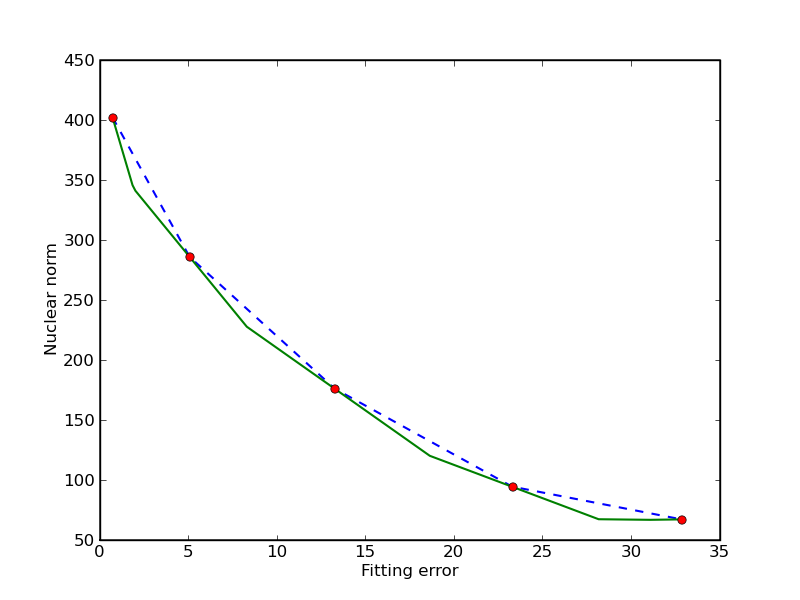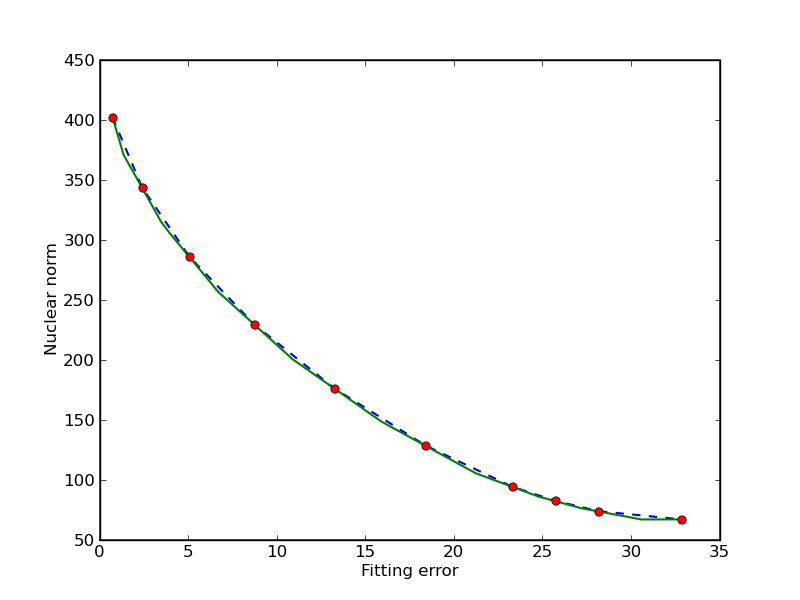# Nuclear norm approximation

This software accompanies the paper Interior-point method for nuclear norm approximation with application to system identification (pdf) by Zhang Liu and Lieven Vandenberghe. The code can be downloaded as a zip file and requires CVXOPT version 1.1.1 or higher and Python 2.7.

## Purpose

A function is provided to solve a linear matrix approximation problem in the nuclear norm, possibly with quadratic regularization. The general optimization problem is

$\begin{split}\begin{array}[t]{ll} \mbox{minimize} & \|A(x) - B\|_* + (1/2) x^T C x + d^T x \\ \mbox{subject to} & Gx \preceq h \end{array}\end{split}$

where

$A(x) = x_1 A_1 + x_2 A_2 + \cdots + x_n A_n$

is a linear matrix valued function. The norm in the first term of the objective is the nuclear norm (sum of singular values). The second term is a general convex quadratic function. The constraints are componentwise linear inequalities.

This problem is of interest as a convex heuristic for a regularized rank minimization problem

$\begin{split}\newcommand{\Rank}{\mathop{\bf rank}} \begin{array}[t]{ll} \mbox{minimize} & \Rank(A(x) - B) + (1/2) x^T C x + d^T x \\ \mbox{subject to} & Gx \preceq h \end{array}\end{split}$

which in general is very difficult.

## System identification example

The package contains several examples, including a system identification problem. In this example, we fit a linear state space model to measured inputs and outputs of a dynamical system. The model is constructed from the solution of a regularized nuclear norm minimization problem

$\begin{array}{ll} \mbox{minimize} & \displaystyle \| Y U^\perp \|_* + \gamma \sum_{t=1}^N \|y(t) - y_\mathrm{meas}(t)\|_2^2. \end{array}$

The optimization variables $$y(t)$$ in the problem are the model outputs. The vectors $$y_\mathrm{meas}(t)$$ are given, measured, outputs. The matrix $$Y$$ is a block Hankel matrix constructed from the variables $$y(t)$$. The matrix $$U^\perp$$ is a matrix whose columns span the nullspace of a block Hankel matrix $$U$$ constructed from measured inputs $$u_\mathrm{meas}(t)$$. At the optimum the matrix $$Y U^\perp$$ is low rank, and its rank is equal to the order of a linear model that maps the measured inputs to the corrected outputs.

The figures illustrate the results for the compact disc arm data of the Daisy collection. The first figure shows the singular values of the matrix $$YU^\perp$$ constructed from the optimized outputs, and the matrix $$Y_\mathrm{meas}U^\perp$$ constructed from the measured outputs. The plot suggests a model of order three. The second figure compares the actual outputs with the outputs of the identified model.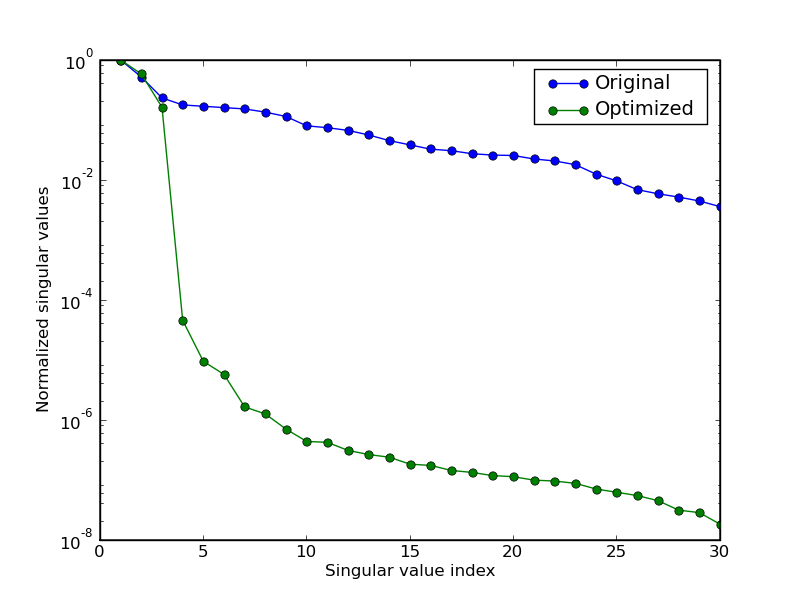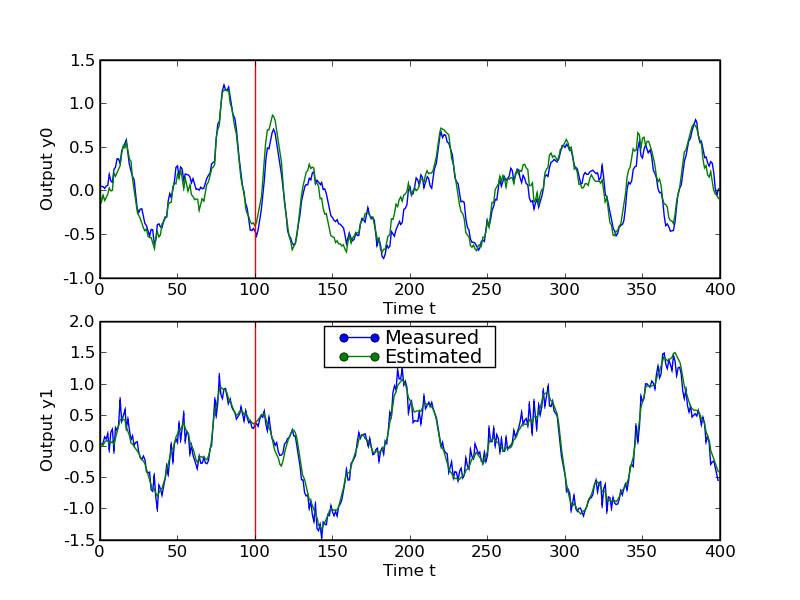$\newcommand{\reals}{\mbox{\bf R}} \begin{array}{ll} \mbox{minimize (w.r.t. \reals_2^+)} & (\|A(x) - B\|_*, \;\; \| x-x_0\|_2^2) \end{array}$
$\begin{array}{ll} \mbox{minimize} & \|A(x) - B\|_* + \gamma \| x-x_0\|_2^2 \end{array}$